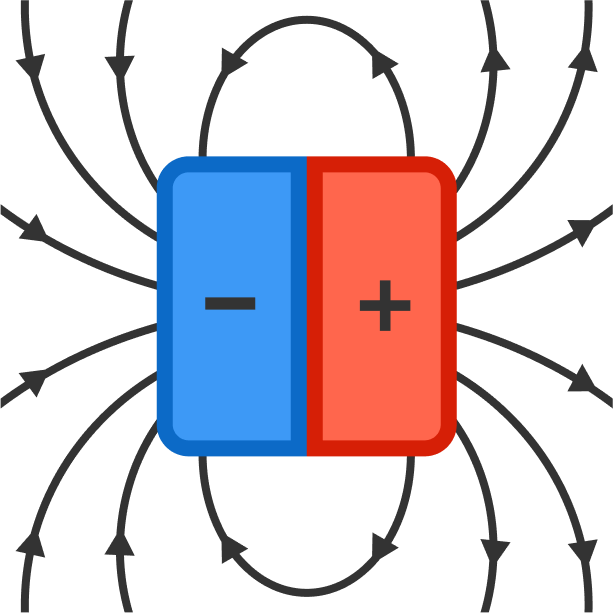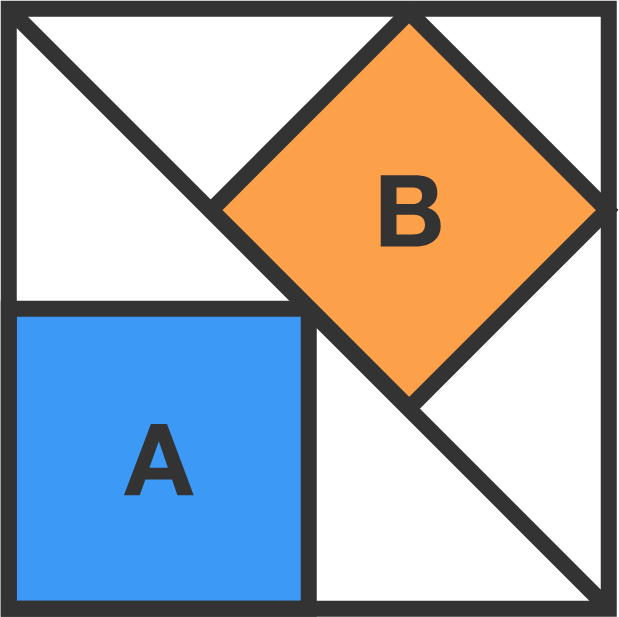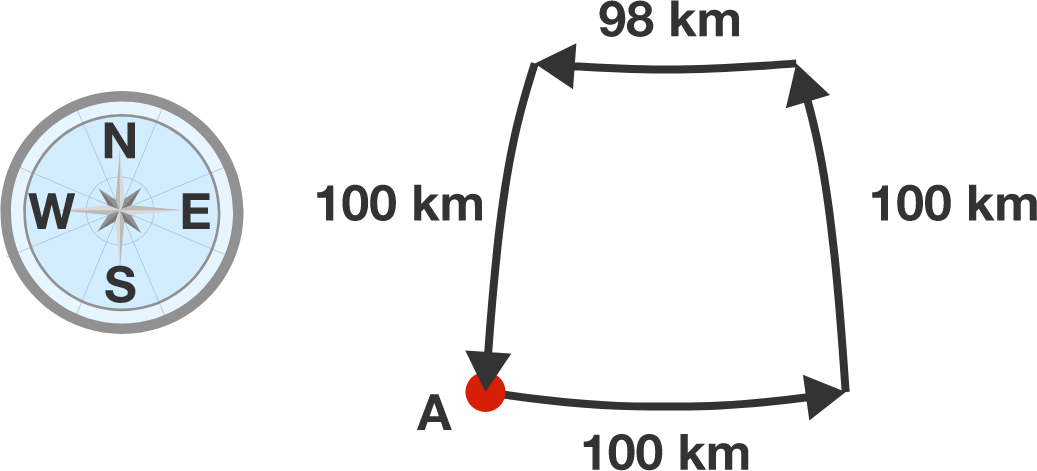# Problems of the Week

Contribute a problem

# 2018-03-26 IntermediateJohnny and Klaus engineer an array of magnets in the following pattern:Is the magnetic field stronger on side A or B?

A diagonal divides a large, outer square into two equal parts. A smaller square is inscribed in each part. Let the area of the blue square be $A,$ and let the area of the orange square be $B.$

What is $\frac AB?$Which is larger?

\begin{aligned} A &= \dfrac1{2} + \dfrac1{2\times2} + \dfrac1{2\times2\times2} + \dfrac1{2\times2\times2\times2} + \cdots \\ \phantom0\\ B &= \dfrac0{2} + \dfrac1{2\times2} + \dfrac2{2\times2\times2} + \dfrac3{2\times2\times2\times2} + \cdots \end{aligned}

A nomadic tribe in the northern hemisphere moves along the following route every year:

• In spring, the tribe moves 100 km to the east.
• In summer, the tribe moves 100 km to the north.
• In autumn, the tribe moves 98 km to the west.
• In winter, the tribe moves 100 km to the south.

The tribe reaches its exact starting point from the spring and sets up its winter quarters there.

At what latitude $\phi$ are the winter quarters (in degrees)? Round to the nearest integer.Note: Earth's radius is $R = 6371 \,\text{km}.$

Lynn has a calculator with only two buttons that perform $\boxed{+1}$ and $\boxed{\div 2}$.

She also has a screen with $9$ significant figures, and it displays $0$ when she gets the calculator.

If she wants to display $\pi$ up to the eighth decimal $(3.14159265)$, what is the fewest number of taps she needs to do?

Hint: The first 64 bits of $\pi$ in binary are given below:

$\pi \approx$11.00100100001111110110101010001000100001011010001100001000110100$_2$

Note: You may want to use the code environment below.

You need to be connected to run code

×

Problem Loading...

Note Loading...

Set Loading...